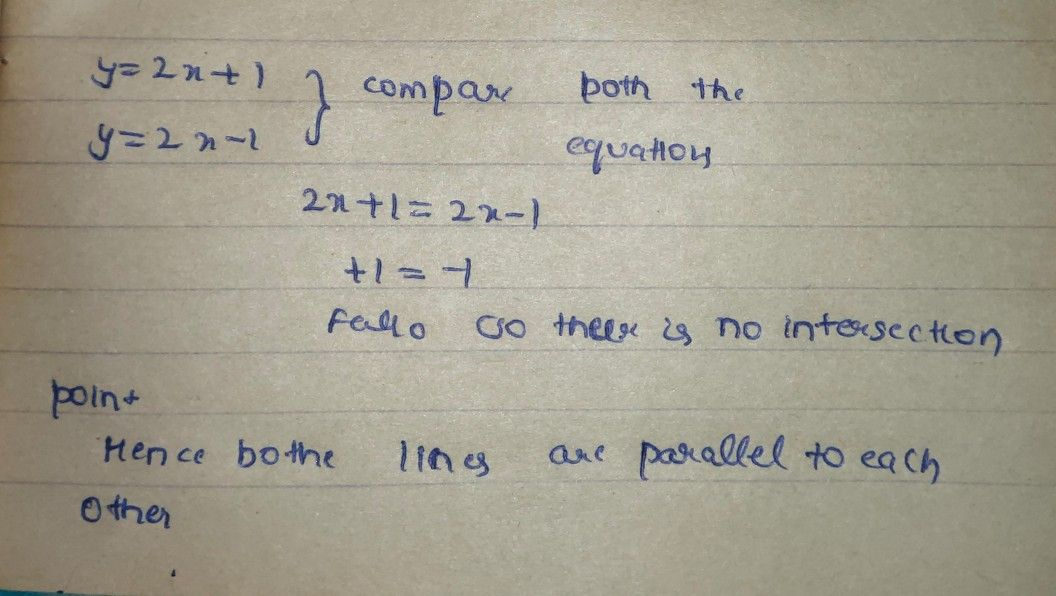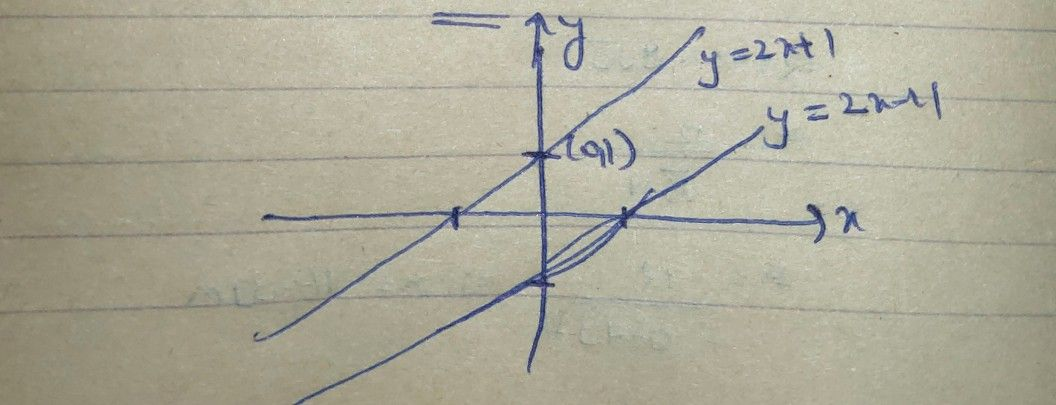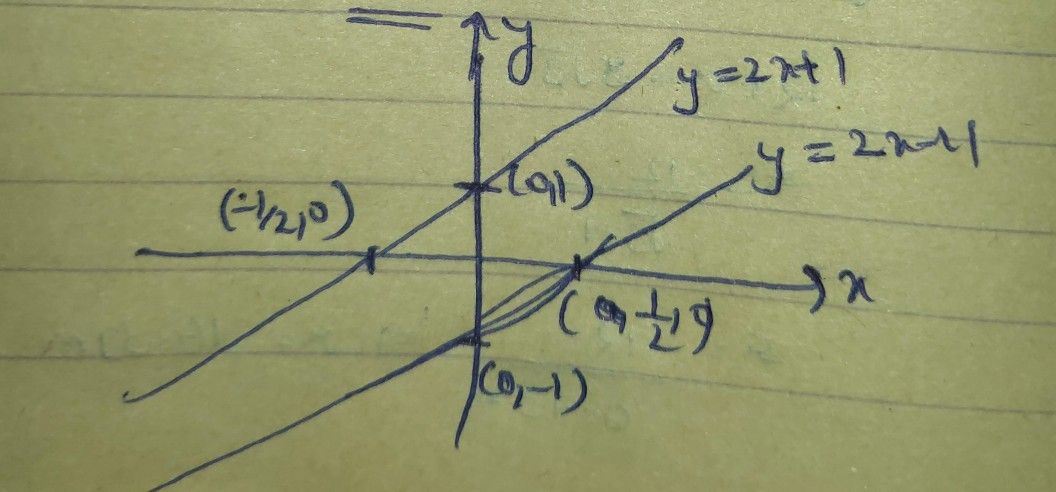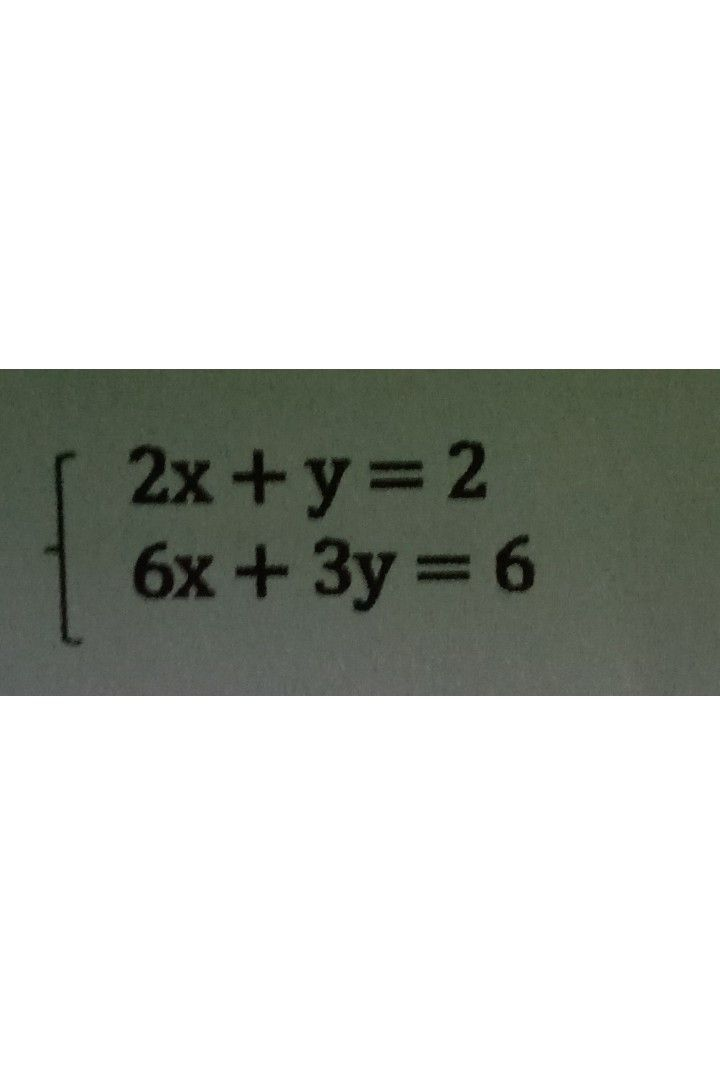Symbol
Problem$Direction$ Graph the given system of linear equations in two variables using any method. $Descr1b6$ the graphs formed and determine the point/s of intersection. Write your answer on the space provided $1. \begin{cases} y=2x+1 \\ y=2x-1 \end{cases}$
7th-9th grade
Other
Search count: 114
SolutionQanda teacher - AskhinaStudent
can you pls put numbers on the side of the graph so it would be easier to understand
are you there teacher?
teacher???Qanda teacher - Askhina
yea
i willStudent
ok thx
may I ask something? can I only ask one question like this?Qanda teacher - Askhina
yes
askStudenthow about this one?Qanda teacher - Askhina
Please ask as preferred matchStudent
what do u mean by preferred match?Qanda teacher - Askhina
Click on my photo
and then ask a questionStudent
it says this teacher does not allow preferred match questionsQanda teacher - Askhina
no please try one more timeStudent
I sent the question
where is the answer? I have an extra 1k coin
have
gave*Qanda teacher - Askhina
i will give already
Please visit another chatStudent
ok